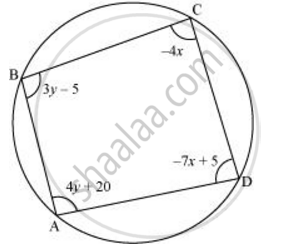# ABCD is a cyclic quadrilateral finds the angles of the cyclic quadrilateral. - Mathematics

ABCD is a cyclic quadrilateral finds the angles of the cyclic quadrilateral.#### Solution

We know that the sum of the measures of opposite angles in a cyclic quadrilateral is 180°.

Therefore, ∠A + ∠C = 180

4y + 20 − 4x = 180

− 4x + 4y = 160

x − y = − 40 (i)

Also, ∠B + ∠D = 180

3y − 5 − 7x + 5 = 180

− 7x + 3y = 180 (ii)

Multiplying equation (i) by 3, we obtain

3x − 3y = − 120 (iii)

Adding equations (ii) and (iii), we obtain

− 7x + 3x = 180 − 120

− 4x = 60

x = −15

By using equation (i), we obtain

− y = − 40

−15 − y = − 40

y = −15 + 40 = 25

∠A = 4y + 20 = 4(25) + 20 = 120°

∠B = 3y − 5 = 3(25) − 5 = 70°

∠C = − 4x = − 4(− 15) = 60°

∠D = − 7x + 5 = − 7(−15) + 5 = 110°

Concept: Equations Reducible to a Pair of Linear Equations in Two Variables
Is there an error in this question or solution?

#### APPEARS IN

NCERT Class 10 Maths
Chapter 3 Pair of Linear Equations in Two Variables
Exercise 3.7 | Q 8 | Page 68